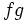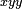# Product rule for higher partial derivatives

## Statement

The general statement is a little complicated, because of the notation used for tracking the combinatorics. The idea is as follows:

• We consider the string with respect to which we are differentiating.
• Consider all ways of dividing this string into two substrings, each of which maintains the same order as the original string. One of the substrings may be empty.
• Now consider a term obtained by differentiating$f$ with respect to the first substring and$g$ with respect to the second substring.
• Add up all these terms. Since the number of substrings is$2^n$ (with$n$ the order of the derivative), we expect a sum of$2^n$ terms. In case there are repetitions in the string being differentiated with respect to, some of the terms may be equal and could be combined.

## Particular cases

Case Statement
second-order mixed partial derivative with respect to$x,y$ of a product$fg$ of functions that involve at least two variables$x,y$$(fg)_{xy} = f_{xy}g + f_xg_y + f_yg_x + fg_{xy}$
derivative of the form${}_{xyy}$ of a product$fg$ of functions that involve at least two variables$x,y$$(fg)_{xyy} = f_{xyy}g + 2f_{xy}g_y + + f_xg_{yy} + 2f_yg_{xy} + f_{yy}g_x + fg_{xyy}$